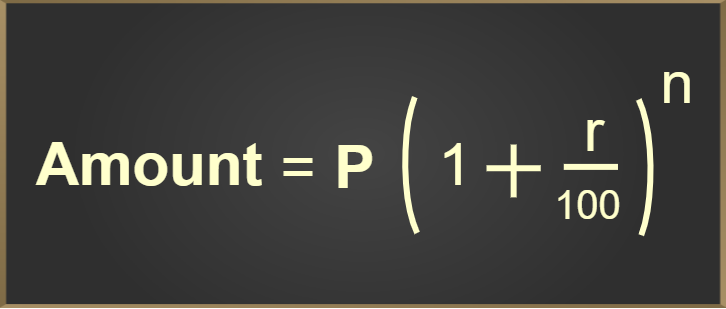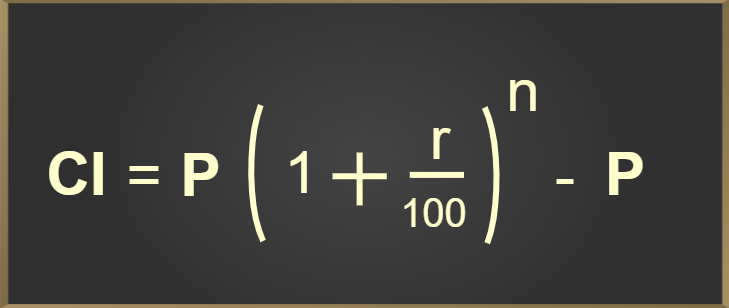GeeksforGeeks App
Open AppBrowser
Continue

# Compound Interest Formula

Compound interest is the interest that is calculated against a loan or deposit amount in which interest is calculated for the principal as well as the previous interest earned. The common difference between compound and simple interest is that in compound interest, interest is calculated for the principal amount as well as for the previously earned interest whereas simple interest depends only on the principal invested.

Simple interest formula is calculated on the principal, suppose principal = p, rate of interest = r, time = t, Then

SI = (p × t × r)/100

Amount: The total sum of money that a person gets after a certain period of time including the interest is called the amount. For Compound Interest the Amount is calculated using the formula given below,Compound interest is calculated on the principal amount and also on the accumulated interest of previous periods.

CI = A – P

## Definition of Compound Interest

Compound interest is the interest calculated on the principal and the interest earned previously. It is denoted by C.I. it is very useful for investment and loan repayment purposes. It is also known as “interest on interest”.

Compound interest is very useful in the banking and finance sectors and is also useful in other sectors. A few of its use are:

• Growth of population of a country
• Value of investment over a period of time.
• For finding Inflated costs and the depreciated value of any article.
• For predicting the growth of any institution or country.

Compound interest (C.I) = Amount – Principal

## How to Calculate Compound Interest?

Compound interest is the interest paid both on principal as well as interest accumulated. The interest earned at each interval is added to the initial principal ad thus principal goes on increasing.

Use the following methods to find the compound interest.

Step 1: Note the Principal, rate, and time period given.

Step 2: Calculate the amount using the formula A = P(1 + r/100)n

Step 3: Find the Compound Interest using the formula CI = Amount – Principal

At regular intervals, the interest so far accumulated is clubbed with the existing principal amount and then the interest is calculated for the new principal. The new principal is equal to the sum of the Initial principal, and the interest accumulated so far.

Compound Interest = Interest on Principal + Compounded Interest at Regular Intervals

The compound interest is calculated at regular intervals like annually(yearly), semi-annually, quarterly, monthly, etc; It is like, re-investing the interest income from an investment makes the money grow faster over time! It is exactly what compound interest does to money. Banks or any financial organization calculate the amount based on compound interest only.

## Compound Interest Formula

Compound interest is calculated, after calculating the total amount over a period of time, based on the rate of interest, and the initial principal. For an initial principal of P, rate of interest per annum of r, time period t in years, frequency of the number of times the interest is compounded annually n, the formula for calculation of CI is as follows.Where,
P = principal
r = rate of interest
n = number of times interest is compounded per year
t = time (in years)

Compound Interest = A – P

Compound Interest can be calculated yearly, half-yearly, quarterly, monthly, daily, etc as per the requirement.

## Half-yearly Compound Interest formula

Let the principal invested be P and the interest rate is R % per annum which is compounded half-yearly for t years

As it is compounded half-yearly, the principal will be changed at the end of 6 months, and interest earned till then will be added to the principal and then this becomes the new principal. Similarly, the final amount is calculated.

we know,

rate = R% per annum compounded half yearly
rate = (R/2)%
time is t years we know that t years have 2t half years

Now,

A = P(1 + R/200)2t

CI = A – P

## Quarterly Compound Interest formula

Let the principal invested be P and the interest rate is R % per annum which is compounded quarterly for t years

As it is compounded quarterly, the principal will be changed at the end of 3 months, and interest earned till then will be added to the principal and then this becomes the new principal. Similarly, the final amount is calculated.

we know,

rate = R% per annum compounded quarterly
rate = (R/4)%
time is t years we know that t years have 4t quarters

Now,

A = P(1 + R/400)4t

CI = A – P

## Periodic Compounding Rate

The total amount, including the principal P and compounded interest CI is given by:

A = P[1 + (r/n)]nt

where,

P = Principal
A = Final amount
r = annual interest rate
n = number of times interest is compounding
t = Time (in years)

Thus, compound interest is:

CI = A – P

## Rule of 72

Rule of 72 is the formula that is used to estimate, how many years our money gets doubled if it is compounded annually. For example, if our money is invested at r % compounded annually then it takes 72/r years for our money to get doubled.

This calculation is also useful for calculating the inflated value of our money, i.e. it gives in how many years the value of our asset gets halved if it gets depreciated annually.

### Rule of 72 formula

The following formula is used to approximate the number of years for our investment to get doubled.

N = 72 / r

where,
N is approximate number of year our money get doubled
r is the rate at which our money is compounded annually

### Rule of 72 example

Suppose Kabir has invested 10,00,000 rupees in a debt fund which gives an 8% return. Find in how many years its money gets doubled if it is compounded annually.

Using above formula: N = 72/8 = 9 years.
Thus, it takes 9 years for Kabir’s money to get doubled.

## Compound Interest of Consecutive Years

If we have the same sum and at the same rate of interest. The C.I of a particular year is always more than C.I of Previous Year. (CI of 3rd year is greater than CI of 2nd year). The difference between CI for any two consecutive years is the interest of one year on C.I of the preceding year.

C.I of 3rd year – C.I of 2nd year = C.I of 2nd year × r × 1/100

The difference between the amounts of any two consecutive years is the interest of one year on the amount of the preceding year.

Amount of 3rd year – Amount of 2nd year = Amount of 2nd year × r × 1/100

### Key Results

When we have the same sum and same rate,

C.I for nth year = C.I for (n – 1)th year + Interest for one year on C.I for (n – 1)th year

## Some Other Applications of Compound Interest

Growth: This is mainly used for growth if industries are related.

Production after n years = initial production × (1 + r/100)n

Depreciation: When the cost of a product depreciates by r% every year, then its value after n years is

Present value × (1 + r/100)n

Population Problems: When the population of a town, city, or village increases at a certain rate per year.

Population after n years = present population × (1 + r/100)n

## Difference between Compound Interest and Simple Interest

The difference between Compound Interest and Simple Interest can be learned below in this article

## Solved Examples on Compound Interest

Example 1: Find the Compound Interest when principal = Rs 6000, rate = 10% per annum and time = 2 years?

Solution:

Interest for first year = (6000 × 10 × 1)/100 = 600

Amount at the end of first year = 6000 + 600 = 6600

Interest for second year = (6600 × 10 × 1) / 100 = 660

Amount at the end of second year = 6600 + 660 = 7260

Compound Interest = 7260 – 6000 = 1260

Example 2: What will be the compound interest on Rs 8000 in two years when the rate of interest is 2% per annum?

Solution:

Given principal P = 8000

rate r = 2%

time = 2years

by formula ,

A = P (1 + R/100)n

= 8000 (1 + 2/100)2

= 8000 (102/100)2

= 8323

Compound interest = A – P

= 8323 – 8000

= Rs 323

Example 3: Hari deposited Rs. 4000 with a finance company for 2 years at an interest of 5% per annum. What is the compound interest that Rohit gets after 2 years?

Solution:

Given

principal P = 4000

rate r = 5%

time = 2years

By formula ,

A = P (1 + R/100)n

= 4000 (1 + 5/100)2

= 4000 (105/100)2

= 4410

Compound Interest = A – P

= 4410 – 4000

= 410

Example 4: Find the compound interest on Rs. 2000 at the rate of 4 % per annum for 1.5 years. When interest is compounded half-yearly?

Solution:

Given,

principal p = 2000

rate r = 4%

time = 1.5 ( i.e 3 half years )

by formula ,

A = P (1 + R/200)2n

=  2000 (1 + 4/200)3

= 2000 (204/200)3

= 2122

Compound Interest = A – P

= 2122 – 2000

= 122

Example 5: What is the compound interest on 10000 for one year at the rate of 20% per annum, if the interest is compounded quarterly?

Solution:

Given,

Principal P = Rs 10000

rate R = 12% (12/4 = 3 % per quarter year)

Time = 1 year (1 × 4 = 4 quarters)

by formula,

A = P (1 + R/100)n

= 10000 (1 + 3/100)4

= 10000 (103/100)4

= 11255

Compound Interest = A – P

= 11255 – 10000

= 1255

Example 6: Find the compound interest at the rate of 5% per annum for 2 years on that principal which in 2 years at the rate of 5% per annum given Rs. 400 as simple interest.

Solution:

Given

Simple interest SI = 400

rate R = 5%

time T = 2 years

by formula,

Simple interest = (P × T × R)/100

P = (SI × 100)/T × R

= (400 × 100)/2 × 5

= 40000/10

= Rs 4000

Rate of Compound Interest = 5%

time = 2 years

by formula ,

A = P (1 + R/100)

= 4000 (1 + 5/100)

= 4410

Compound Interest = A – P

= 4410 – 4000

= 410

Example 7: Find the compound interest on Rs 30000 at 7% interest compounded annually for two years.

Solution:

Given,

Principal P = Rs 30000

rate R = 7%

Time = 2 year

by formula,

A = P (1 + R/100)n

= 30000 (1 + 7/100)2

= 30000 (107/100)2

= 34347

Compound Interest = A – P

= 34347 – 30000

= 4347

## FAQs on Compound Interest

Question 1: What is the definition of Compound interest?

If the interest is calculated on the principal as well as the previous interest earned over a fixed period of time it is called Compound Interest.

Question 2: How to calculate compound interest?

To calculate Compound Interest first final amount is calculated then it is subtracted from the principal to get the final Compound Interest. The amount is calculated using the formula,

A = P(1 + R/100)t

CI = A – P

where,
A is Amount
P is Principal
R is rate of interest
T is time period
CI is compound interest

Question 3: Is compound interest better than simple interest for investors?

Yes, Compound Interest is far better than Simple Interest for investors.

Question 4: What is the compound interest formula if it is compounded daily?